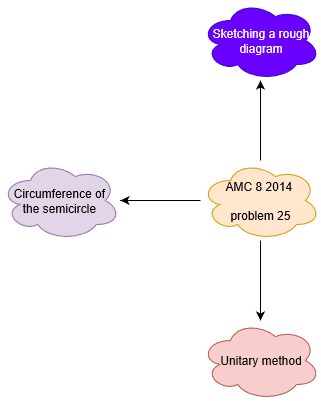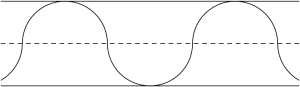# What are we learning ?

Competency in Focus:Geometry of circles. This problem from American Mathematics contest (AMC 8, 2014) is based on simple counting of semicircles.

# First look at the knowledge graph.# Next understand the problem

A straight one-mile stretch of highway, 40 feet wide, is closed. Robert rides his bike on a path composed of semicircles as shown. If he rides at 5 miles per hour, how many hours will it take to cover the one-mile stretch?
Note: 1 mile = 5280feet##### Source of the problem
American Mathematical Contest 2014, AMC 8 Problem 25
##### Key Competency
Geometry of circles
4/10
##### Suggested Book
Challenges and Thrills in Pre College Mathematics Excursion Of Mathematics

Do you really need a hint ? Try it first!
How many lanes the highway consists of ? 2 right! given highway is 40 feet wide .Then width of each lane will be 40/2=20 feet wide .
Look at the diagram .See that the radius of each semicircle will be 20 feet on which Robert must be riding his bike .Again see that each semicrcle covers 40 feet of highway i.e. the diameter of the semicircle .
Calculate the number of semicircles over the whole mile . Number of semicircles=(length of the highway covered in total by Robert)/(length of highway covered by each semicircle)=5280/40 [since 1 mile=5280 feet] =132.
Where the semicircles full circles ,their circumference would be 2.$\pi$ r =2.$\pi$.20=40 $\pi$ feet (since r=radius=20 feet). Therefore the circumference of semicircles is half that, or 20.$\pi$ feet.
Therefore over the stretch of hghway, Robert rides a total of 132.20.$\pi$=2640. $\pi$ feet equivalent to $\frac{ \pi}{2}$  mile( since 1 mile=5280 feet) Given Robert rides at 5 miles per hour.So, time required by Robert =distance travelled/rate=($\frac{\pi}{2}$ miles)/(5 miles per hour)= $\frac{\pi}{10}$hours.

# Connected Program at Cheenta

#### Amc 8 Master class

Cheenta AMC Training Camp consists of live group and one on one classes, 24/7 doubt clearing and continuous problem solving streams.

# Similar Problems

## Median of numbers | AMC-10A, 2020 | Problem 11

Try this beautiful problem from Geometry based on Median of numbers from AMC 10A, 2020. You may use sequential hints to solve the problem.

## The area of trapezoid | AMC 8, 2003 | Problem 21

Try this beautiful problem from Geometry: The area of a trapezoid from AMC-8 (2003). You may use sequential hints to solve the problem.

## Largest area Problem | AMC 8, 2003 | Problem 22

Try this beautiful problem from AMC 8, 2003, problem no-22 based on Largest area. You may use sequential hints to solve the problem.

## Triangle and Quadrilateral | AMC-10A, 2005 | Problem 25

Try this problem from Geometry: Ratios of the areas of Triangle and Quadrilateral from AMC-10A, 2005 You may use sequential hints to solve the problem.

## AP GP Problem | AMC-10A, 2004 | Question 18

Try this beautiful problem from Algebra based on AP GP from AMC-10A, 2004. You may use sequential hints to solve the problem.

## Probability in Divisibility | AMC-10A, 2003 | Problem 15

Try this beautiful problem from AMC 10A, 2003 based on Probability in Divisibility. You may use sequential hints to solve the problem.

## Area of the Region Problem | AMC-10A, 2007 | Problem 24

Try this beautiful problem from Geometry: Area of region from AMC-10A, 2007, Problem-24. You may use sequential hints to solve the problem.

## Circular Cylinder Problem | AMC-10A, 2001 | Problem 21

Try this beautiful problem from Geometry: circular cylinder from AMC-10A, 2001. You may use sequential hints to solve the problem.

## Problem on Circumscribed Circle | AMC-10A, 2003 | Problem 17

Try this beautiful problem from Geometry:Radius of a circle.AMC-10A, 2003. You may use sequential hints to solve the problem

## Problem on Positive Integers | PRMO-2019 | Problem 26

Try this beautiful problem from Algebra based on positive integers from PRMO 2019. You may use sequential hints to solve the problem.